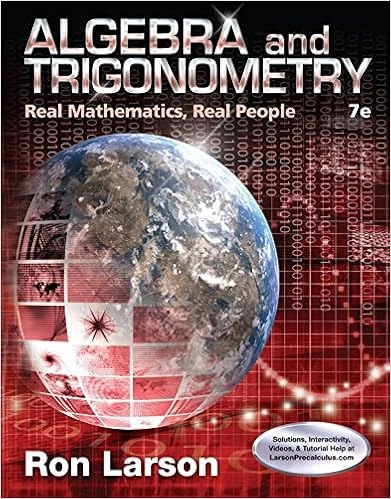# Linear Algebra with Applications (3rd Edition)

• Homework Help
• davidvictor
• 9
• 100% (2) 2 out of 2 people found this document helpful

This preview shows page 1 - 4 out of 9 pages.

##### We have textbook solutions for you!
The document you are viewing contains questions related to this textbook.The document you are viewing contains questions related to this textbook.
Chapter 4 / Exercise 63
Algebra and Trigonometry: Real Mathematics, Real People
LarsonExpert Verified
THE JOHNS HOPKINS UNIVERSITY Faculty of Arts and Sciences FINAL EXAM - SPRING SESSION 2005 110.201 - LINEAR ALGEBRA. Examiner: Professor C. Consani Duration: 3 HOURS (9am-12noon), May 12, 2005. No calculators allowed. Total Marks = 100 Student Name : TA Name & Session : 1. 2. 3. 4. 5. 6. 7. 8. Total
##### We have textbook solutions for you!
The document you are viewing contains questions related to this textbook.The document you are viewing contains questions related to this textbook.
Chapter 4 / Exercise 63
Algebra and Trigonometry: Real Mathematics, Real People
LarsonExpert Verified
1. [10 marks] Consider the matrix A = 2 1 0 4 2 1 1 2 4 2 3 2 . 1a. [2 marks] Compute the reduced row-echelon form of A . 1b. [2 marks]Determine the rank ofA. 1c. [2 marks]Determine a basis of the column space ofA. 1d. [2 marks]Determine a basis of the nullspace ofA. 1e. [2 marks] For what value(s) of r R is the following system solvable Ax = 2 3 r ? Sol. [1a.] A = 2 1 0 4 2 1 1 2 4 2 3 2 2 1 0 4 0 0 1 - 2 4 2 3 2 2 1 0 4 0 0 1 - 2 0 0 3 - 6 2 1 0 4 0 0 1 - 2 0 0 0 0 1 1 / 2 0 2 0 0 1 - 2 0 0 0 0 rref( A ) = 1 1 / 2 0 2 0 0 1 - 2 0 0 0 0 . [1b.] It follows from the description of rref( A ) that rk( A ) = 2. [1c.] A basis of the column space of A is given by the vectors 2 2 4 and 0 1 3 corre- sponding to the pivot columns in A . [1d.] There are two non-trivial relations among the columns of A . Let denote by v 1 , . . . , v 4 the first,...,fourth column of A . We have v 1 - 2 v 2 = 0 , 2 v 1 - 2 v 3 - v 4 = 0 . Hence, a basis of the nullspace of A is given by the vectors 1 - 2 0 0 and 2 0 - 2 - 1 . [1e.] Consider the complete matrix B associated to the system B = 2 1 0 4 | 2 2 1 1 2 | 3 4 2 3 2 | r 2 1 0 4 | 2 0 0 1 - 2 | 1 0 0 3 - 6 | r - 4 2 1 0 4 | 2 0 0 1 - 2 | 1 0 0 0 0 | r - 7 The system is solvable if and only if r = 7.
2. [15 marks] Consider the matrix A = 1 4 1 1 1 1 . 2a. [5 marks] Give a factorization A = QR , where R is an upper-triangular matrix and Q is a matrix with orthonormal columns. 2b. [5 marks] Find the least square solution to the system Ax = b , for b = 4 8 6 . 2c. [5 marks] The projection matrix P = A ( A T A ) - 1 A T projects all vectors onto the column space of A . Find a vector q , not in the column space of A such that Pq = 1 4 4 . Sol. [2a.] Perform Gram-Schmidt process on the (linearly independent) columns of A and get two orthonormal vectors fitting the columns of Q = 1 3 2 6 1 3 - 1 6 1 3 - 1 6 . Then, obtain R via R = Q T A , i.e. R = 3 2 3 0 6 .
•••